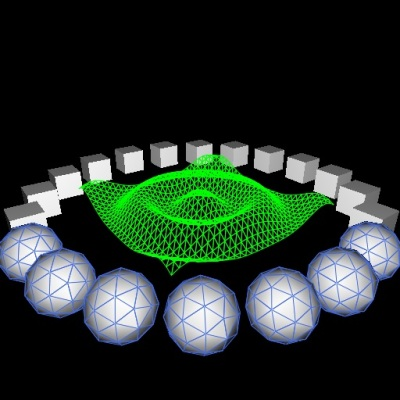Visualization Library 2.1.0 A lightweight C++ OpenGL middleware for 2D/3D graphics VL     Star     Watch     Fork     Issue [Download] [Tutorials] [All Classes] [Grouped Classes]
Geometry LOD and Animation

In this tutorial you will learn how to define a set of LOD (level of detail) geometries using the vl::DistanceLODEvaluator class and how to animate your geometry using the vl::ActorEventCallback class.This demo creates a scene containing a central animated wave geometry and a few objects surrounding it in a circle. The central wave geometry defines 3 level of detail which will be animated in realtime using a subclass of vl::ActorEventCallback created ad-hoc. The surrounding objects are there to demonstrate even more clearly how to use geomety LOD. Each objects is rendered as a sphere at LOD level #0, as a cube at LOD level #1 and as a wireframe pyramid at LOD level #2. Note that the demo also uses the same LOD evaluator to perform Effect lod, demonstrating how simple it is to synchronize geometry and effect LOD.

[From `App_GeomLODAnim.cpp`]

#include "BaseDemo.hpp"
/*
Implements an animated wave geometry.
*/
class WaveActorAnimator: public vl::ActorEventCallback
{
public:
// initialize the 3 gometry LODs and installs them into the specified Actor
WaveActorAnimator(vl::Actor* actor)
{
mLastUpdate = 0;
mLastUpdatedLod = NULL;
const float side = 40;
const int detail = 60;
// LOD 0
geom = vl::makeGrid( vl::vec3(0,0,0), side, side, detail, detail );
// geom->setColor(vl::royalblue);
actor->setLod(0, geom.get());
if (vl::Has_GL_ARB_vertex_buffer_object)
{
}
// LOD 1
geom = vl::makeGrid( vl::vec3(0,0,0), side, side, detail/2, detail/2 );
// geom->setColor(vl::green);
actor->setLod(1, geom.get());
if (vl::Has_GL_ARB_vertex_buffer_object)
{
}
// LOD 2
geom = vl::makeGrid( vl::vec3(0,0,0), side, side, detail/4, detail/4 );
// geom->setColor(vl::yellow);
actor->setLod(2, geom.get());
if (vl::Has_GL_ARB_vertex_buffer_object)
{
}
}
// respond to onActorRenderStarted() event by animating the wave
virtual void onActorRenderStarted(vl::Actor*, vl::real frame_clock, const vl::Camera*, vl::Renderable* renderable, const vl::Shader*, int pass)
{
/* the beauty of this function is that in a few lines of code we update 3 different LOD levels! */
// update the geometry only the first time it is drawn
if (pass > 0)
return;
// clamp animation to 30 FPS
const vl::real fps = 30.0f;
if ( frame_clock - mLastUpdate > 1.0f/fps || mLastUpdatedLod != renderable )
{
mLastUpdate = frame_clock;
mLastUpdatedLod = renderable;
// note: this returns the current LOD geometry
vl::ref<vl::Geometry> geom = vl::cast<vl::Geometry>( renderable );
vl::ref<vl::ArrayFloat3> vecarr3 = vl::cast<vl::ArrayFloat3>( geom->vertexArray() );
vl::fvec3* vec = vecarr3->begin();
vl::vec3 center = renderable->boundingBox().center();
float phi = 0.5;
float theta = 1.5;
for(size_t i=0; i<vecarr3->size(); ++i)
{
// flatten to xz plane and compute distance
vec[i].y() = 0;
vl::real d = (vl::vec3(vec[i])-center).length();
vec[i].y() = (float)cos( -frame_clock * vl::fPi * theta + d * phi ) * 2.0f;
}
if (vl::Has_GL_ARB_vertex_buffer_object)
{
}
// when modifying the vertices of a geometry always remember to update the bounding volumes!
geom->setBoundsDirty(true);
}
}
// don't respond to onActorDelete() event.
virtual void onActorDelete(vl::Actor*) { }
protected:
vl::real mLastUpdate;
vl::Renderable* mLastUpdatedLod;
};
/*
This test shows how to enable shader-lod, geometry-lod and how to animate a simple wave geometry over different LODs.
*/
class App_GeomLODAnim: public BaseDemo
{
public:
void initEvent()
{
vl::Log::notify(appletInfo());
/* configure how many objects are there forming the ring */
const int ring_obj_count = 20;
/* define a LOD evaluator with 3 disance ranges:  --- 70 --- 150 --- [inf] */
lod_eval->distanceRangeSet().push_back(70);
lod_eval->distanceRangeSet().push_back(150);
/* to be used later */
/* fill pass */
fill_sh->gocMaterial()->setFrontDiffuse( vl::white );
fill_sh->gocPolygonMode()->set(vl::PM_FILL, vl::PM_FILL); // note this is default
fill_sh->setRenderState( light.get(), 0 );
/* wire pass */
wire_sh->enable(vl::EN_BLEND); // for line smoothing
wire_sh->gocMaterial()->setFlatColor( vl::royalblue );
wire_sh->gocPolygonOffset()->set(-1.0f, -1.0f);
wire_sh->setRenderState( light.get(), 0 );
/* wave pass */
wave_sh->enable(vl::EN_BLEND); // for line smoothing
/* animated wave actor has a single Effect LOD */
wave_fx->setLOD( 0, wave_sh.get() );
/* add wave actor */
vl::ref<vl::Actor> wave_act = sceneManager()->tree()->addActor(NULL, wave_fx.get(), NULL);
/* install actor animation callback. */
wave_act->actorEventCallbacks()->push_back( new WaveActorAnimator(wave_act.get()) );
/* install the LOD evaluator */
wave_act->setLODEvaluator(lod_eval.get());
/* effect used by the objects forming a ring around the central wave */
ring_fx->setLOD( 0, fill_sh.get(), wire_sh.get() ); // LOD 0: pass #1 = fill_sh + pass #2 = wire_sh
ring_fx->setLOD( 1, fill_sh.get() ); // LOD 1: pass #1 = fill_sh
ring_fx->setLOD( 2, wire_sh.get() ); // LOD 2: pass #1 = wire_sh
/* install the LOD evaluator to be used with the ring objects */
ring_fx->setLODEvaluator(lod_eval.get());
/* ring element lod #0 */
geom_0->computeNormals();
/* ring element lod #1 */
vl::ref<vl::Geometry> geom_1 = vl::makeBox(vl::vec3(0,0,0),6,6,6);
geom_1->computeNormals();
/* ring element lod #2 */
geom_2->computeNormals();
/* generate the ring of objects */
for(int i=0;i<ring_obj_count; i++)
{
/* define actor position and add it to the scene */
vl::real t = 360.0f / ring_obj_count * i;
vl::vec3 v = vl::mat4::getRotation(t,0,1,0) * vl::vec3(35,0,0);
rendering()->as<vl::Rendering>()->transform()->addChild(tr.get());
vl::ref<vl::Actor> act = sceneManager()->tree()->addActor( NULL, ring_fx.get(), tr.get() );
/* define which geometry to use for each LOD */
act->setLod(0, geom_0.get());
act->setLod(1, geom_1.get());
act->setLod(2, geom_2.get());
/* install the LOD evaluator*/
act->setLODEvaluator(lod_eval.get());
}
}
void updateScene()
{
/* animate the camera to rotate around the scene and bounce near/far */
float s = sin( vl::Time::currentTime() * vl::fPi * 2.0f / 10.0f );
vl::real t = pow((s+1.0f)/2.0f,2);
vl::real x = t * 200 + 5;
vl::vec3 eye( x, 0, 0 );
eye = vl::mat4::getRotation( vl::Time::currentTime() * 30.0f, 0, 1, 0 ) * eye;
eye += vl::vec3(0,10+70*t,0);
m = vl::mat4::getLookAt( eye, vl::vec3(0,0,0), vl::vec3(0,1,0) );
rendering()->as<vl::Rendering>()->camera()->setViewMatrix(m);
}
};
// Have fun!